1687字，阅读需时6分钟1 X 0 = 0

1 X 1 = 1

……

1 X n = n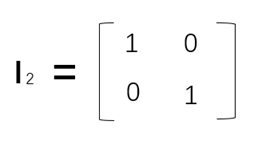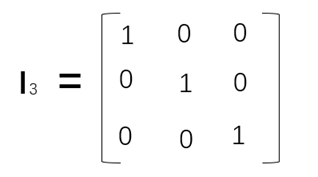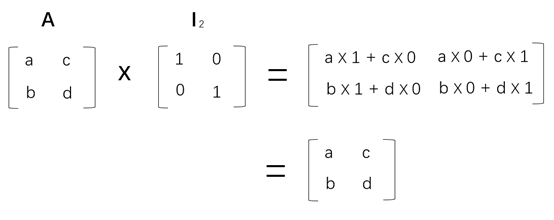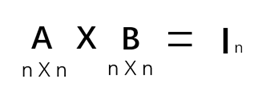A的逆矩阵被记为：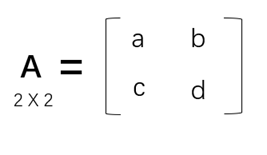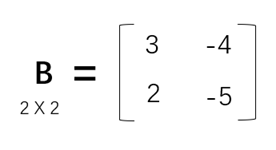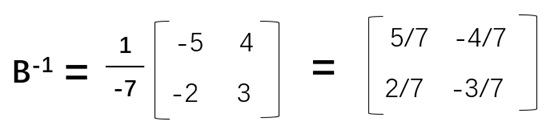B矩阵的行列式计算结果为-7，也就是-1/7乘以B变换后的矩阵，标量与矩阵相乘的规则是标量乘以矩阵的每个元素。B矩阵的逆矩阵为：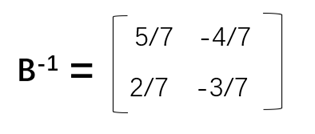```import numpy as np
#定义矩阵B
B = np.array([[3,-4],[2,-5]])

#定义求逆矩阵函数
def matrixInverse(A):
#NumPy的matmul完成矩阵乘法运算
C = np.linalg.inv(A)
return C

#定义矩阵乘法运算函数
def matrixMul(A, B):
#NumPy的matmul完成矩阵乘法运算
C = np.matmul(A,B)
return C
if __name__ == '__main__':
#求B的逆矩阵
C = matrixInverse(B)
print(C)

#验证B的逆矩阵
print(matrixMul(B,C))```